### Hausdorff Point Convolution with Geometric Priors

SCIENCE CHINA Information Sciences 2021

Liqiang Lin1    Pengdi Huang1    Fuyou Xue1    Kai Xu2    Daniel Cohen-Or1,3    Hui Huang1*

1Shenzhen University    2National University of Defense Technology    3Tel-Aviv University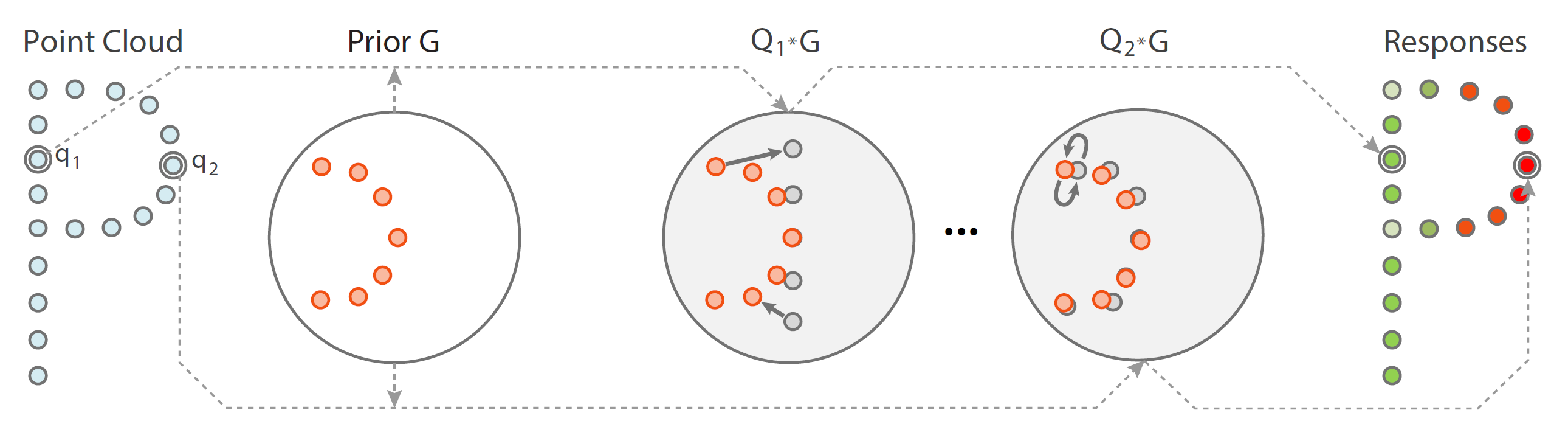Figure 1. In Hausdorff Point Convolution, the response of a local neighborhood (Q1 or Q2) to a geometric kernel (G) is computed based on Hausdorff distance, which is a shape-aware distance measure. Such shape-aware convolution facilitates a powerful point feature learning with a compact set of geometric priors as convolutional kernels.

Abstract

Developing point convolution for irregular point clouds to extract deep features remains challenging. Current methods evaluate the response by computing point set distances which account only for the spatial alignment between two point sets, but not quite for their underlying shapes. Without a shape-aware response, it is hard to characterize the 3D geometry of a point cloud efficiently with a compact set of kernels. In this paper, we advocate the use of modified Hausdorff distance as a shape-aware distance measure for calculating point convolutional responses. The technique we present, coined Hausdorff Point Convolution (HPC), is shape-aware. We show that HPC constitutes a powerful point feature learning with a rather compact set of only four types of geometric priors as kernels. We further develop a HPC-based deep neural network (HPC- DNN). Task-specific learning can be achieved by tuning the network weights for combining the shortest distances between input and kernel point sets. We also realize hierarchical feature learning by designing a multi-kernel HPC for multi-scale feature encoding. Extensive experiments demonstrate that HPC-DNN outperforms strong point convolution baselines (e.g., KPConv), achieving 2.8% mIoU performance boost on S3DIS  and 1.5% on SemanticKITTI  for semantic segmentation task.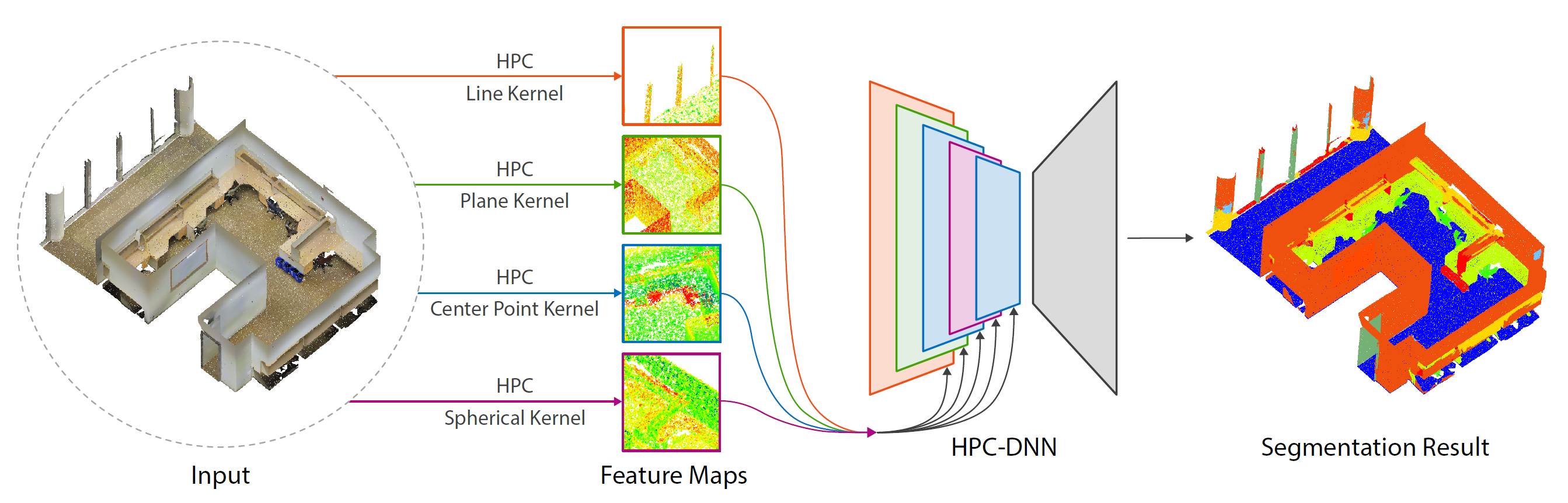Figure 2. An overview of the proposed HPC-DNN. The HPC-DNN adopts a multi-kernels Hausdorff based point convolution (HPC), and extracts hierarchical features from the large-scale input point cloud scene. Then, multi-scale convolution responses of different kernels are merged in each layer to implement scene semantic segmentation.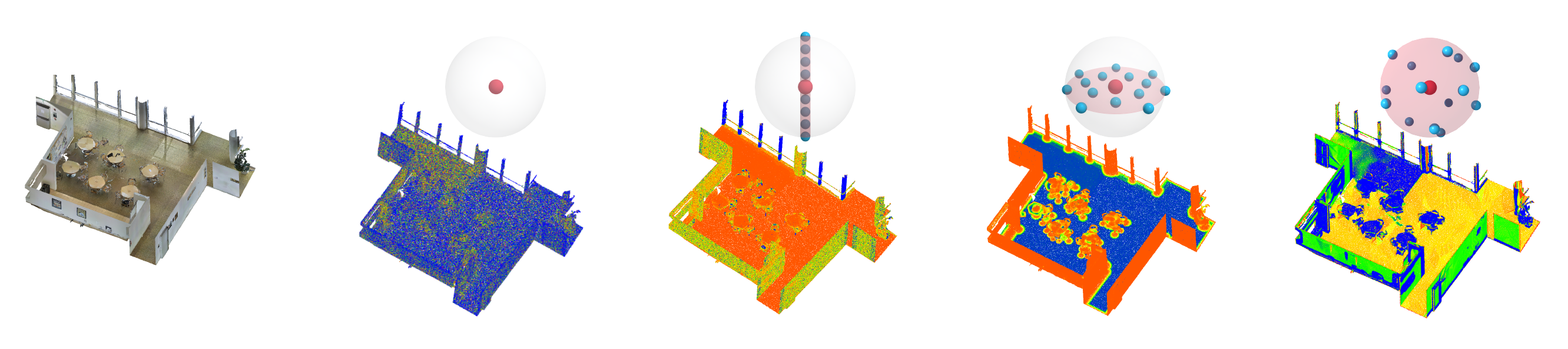Figure 3. Hausdorff distance metric on an indoor scene. The leftmost demonstrates an input scene, while the four images on the right show the four kernels and the corresponding Hausdorff distance responses on the input scene, respectively. The point color (dark to bright) indicates the Hausdorff distance value (low to high) between neighborhood points and the given kernel.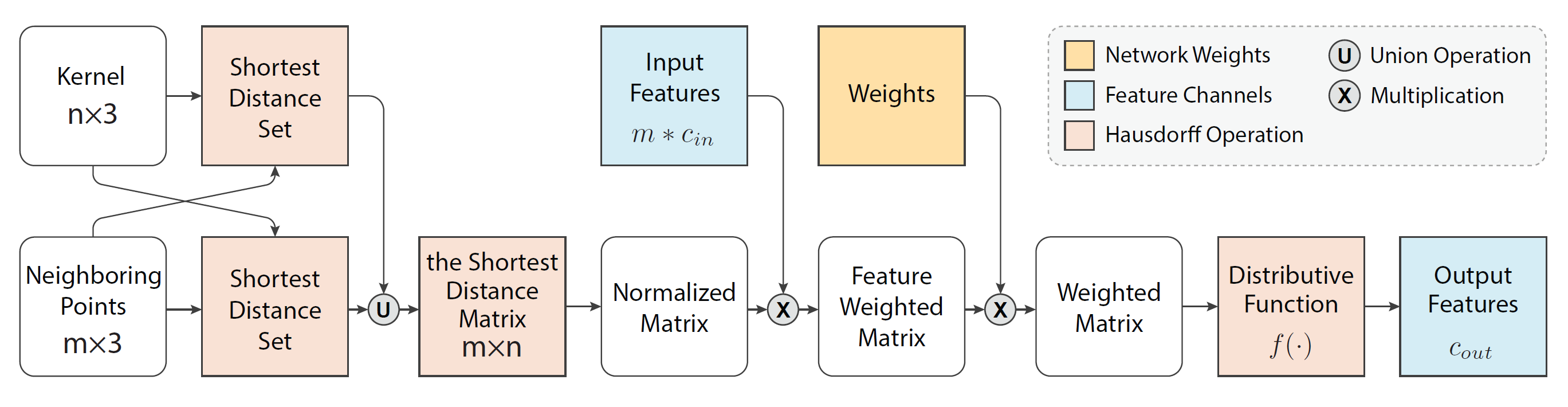Figure 4. A point convolutional layer of the proposed HPC-DNN. The inputs are a query point and its neighboring points with corresponding features and a given kernel points, the output is a feature vector of query point.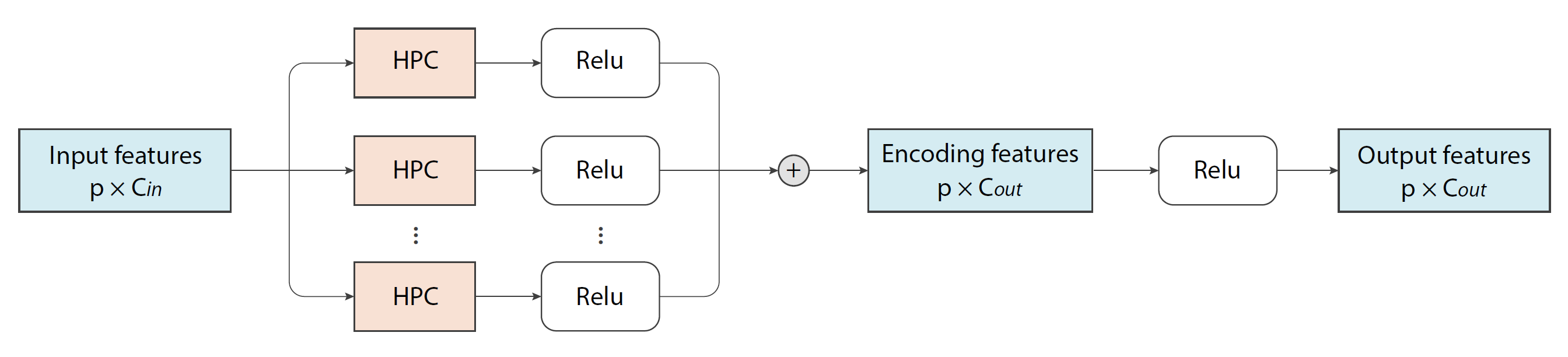Figure 5. The architecture of multi-kernel Hausdorff point convolution layer. For a query point and its input feature Fin, output feature Fout, the convolution results cout of K kernels are linearly accumulated.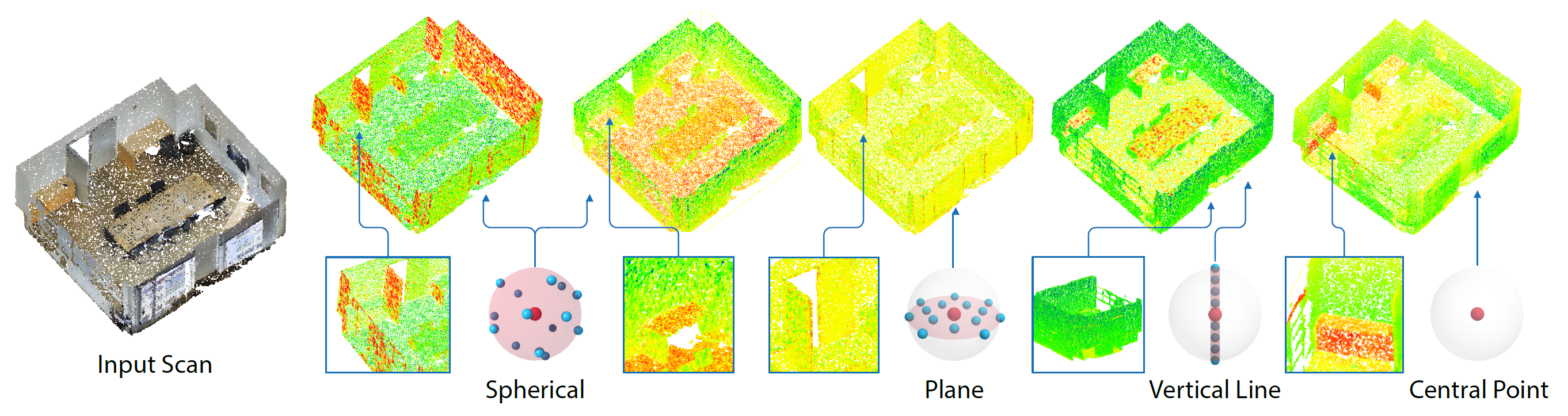Figure 6. Visualization of feature maps on point clouds after point convolution with different kernels. The redder color indicates a higher convolution response after HPC network layer, which fuses the geometric features, input features and network weights.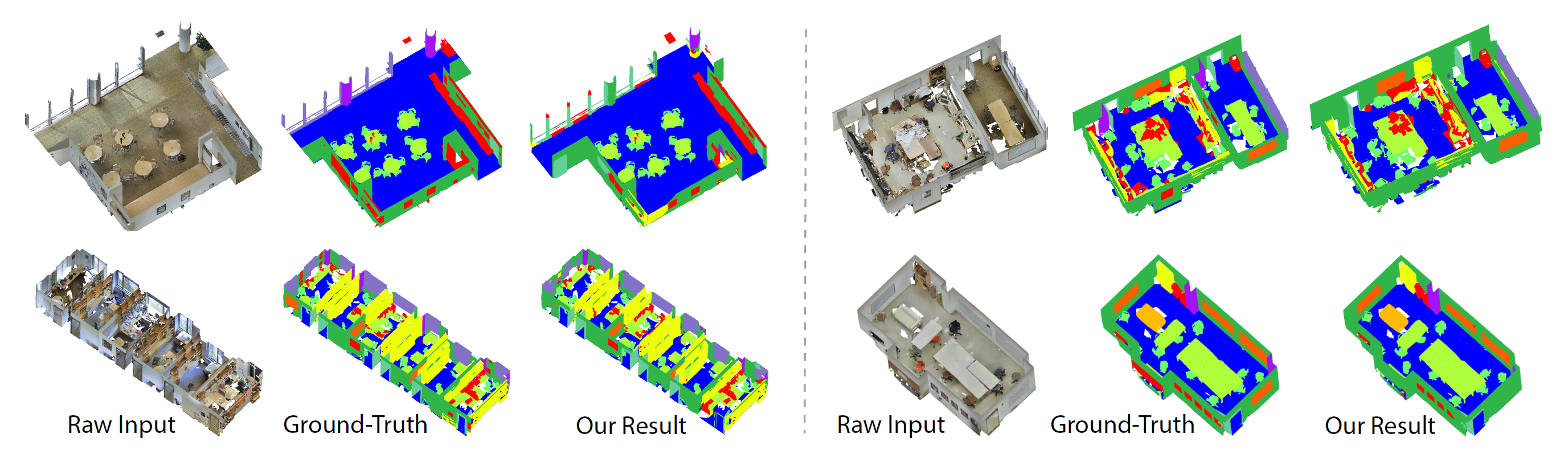Figure 7. A gallery of HPC-DNN segmentation results on the S3DIS dataset.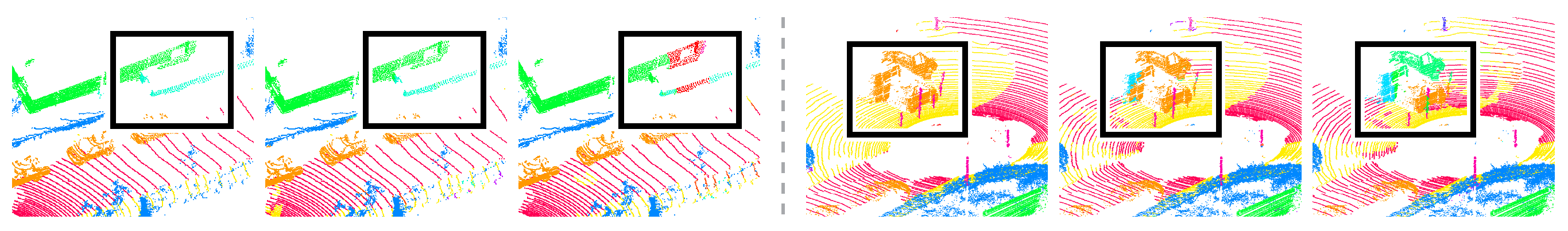Figure 8. Figure A: Visual comparison on SemanticKitti dataset: ground-truth (left), ours (middle), KPConv (right).

Acknowledgements

This work was supported by NSFC (U2001206, 61902254), Guangdong Talent Program (2019JC05X328), Guangdong Science and Technology Program (2020A0505100064, 2015A030312015), DEGP Key Project (2018KZDXM058), Shen- zhen Science and Technology Program(RCJC20200714114435012), National Engineering Laboratory for Big Data System Computing Technology, and Guangdong Laboratory of Artificial Intelligence and Digital Economy (SZ).

Bibtex

@article{HPC21,

title={Hausdorff Point Convolution with Geometric Priors},

author={Liqiang Lin and Pengdi Huang and Fuyou Xue and Kai Xu and Daniel Cohen-Or and Hui Huang},

journal={SCIENCE CHINA Information Sciences},

volume={},

number={},

pages={},

year={2021},

}